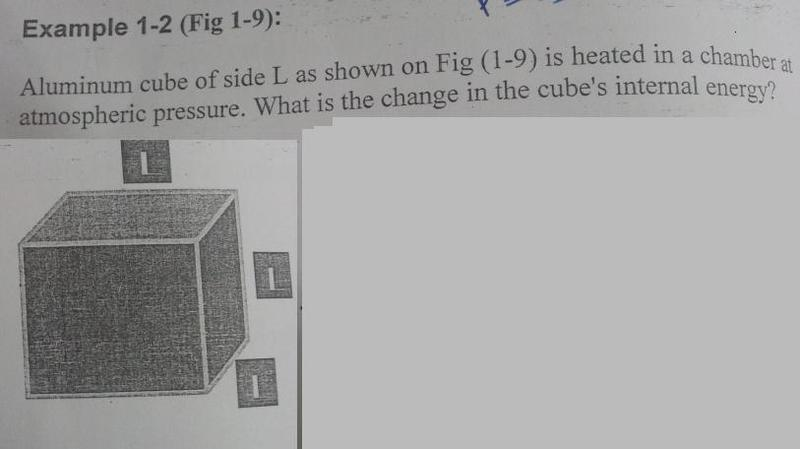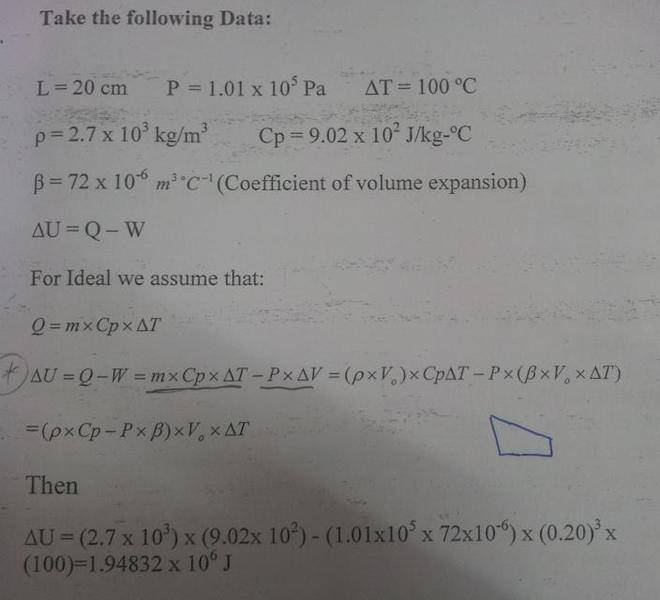# Thermodynamics - example with answer

• manal950

#### manal950

Hi
Here is a question with answer , in fact I don't understand the answer fully ..

and here in answer I saw that work is negative is that because work done by the system ?

The question : -It is based on the 1st law of TD which relates that the energy of the system is conserved.
If Q is the (+) heat that it receives then this energy can be used to raise the internal energy of the system ΔU (+) and/or to do work W (+) thus

Q = ΔU + W

so that

ΔU = Q - W

that is the difference in energy between the received heat and the work done by the system is used to raise its internal energy. In this the aluminium cube does positive work since it is expanding against atmospheric pressure.

Here - W (negative )
Is because the work done by the system during heating ?

and why delta T in C why not in kelvin (K)

thank you

Last edited:
The aluminium does positive work in expanding against the atmospheric pressure, that is the surface exerts an outwards force against the air as it expands. So the direction of movement (expansion) is in the same direction as the force it is exerting, so one inserts it as positive work in the formula

ΔU = Q - W

Weh ndifference of temperature are involved you need not change the temperature to kelvin since their is just a constant difference between the two 273 degrees.

The system will loose energy if it does work, like the expanding block pushing outwards against the air. Because the force that it is exerting against the air is directed outwards and the surface is also moving in this direction, outwards, so it is doing positive work. If the block was cooled down it would still need to support itself against the atmosphere, so it is still pushing back against it, but now the direction of motion is inwards as it is contracting while it is cooling, but now the work done by it is negative since the force and direction of motion are opposite to each other. Also since the atmosphere is pushing it inwards we get that the block will gain energy because the atmosphere is doing work on it. So positive work means it is losing energy and negative work means it is gaining energy.

thanks a lot